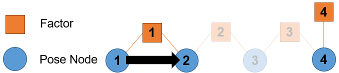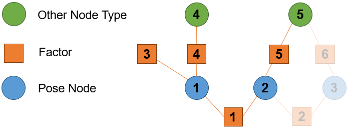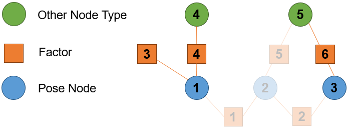# optimize

Optimize factor graph

Since R2022a

## Syntax

``solnInfo = optimize(graph)``
``solnInfo = optimize(graph,poseNodeIDs)``
``solnInfo = optimize(___,solverOptions)``

## Description

The `optimize` function optimizes a factor graph to find a solution that minimizes the cost of the nonlinear least squares problem formulated by the factor graph.

````solnInfo = optimize(graph)` optimizes the factor graph using default solver options, sets the node states to the optimized states, and returns the resulting solution information.```
````solnInfo = optimize(graph,poseNodeIDs)` optimizes the specified pose nodes and any related nodes except for unspecified pose nodes. NoteSpecified node IDs form a connected factor graph. For more information, see Factor Graph Connectivity. ```

example

````solnInfo = optimize(___,solverOptions)` optimizes the factor graph using the specified factor graph solver options, in addition to any combination of input arguments from previous syntaxes.```

## Examples

collapse all

Create a factor graph.

`fg = factorGraph;`

Define two approximate pose states of the robot.

```rstate = [0 0 0; 1 1 pi/2];```

Define the relative pose measurement between two nodes from the odometry as the pose difference between the states with some noise. The relative measurement must be in the reference frame of the second node so you must rotate the difference in position to be in the reference frame of the second node.

```posediff = diff(rstate); rotdiffso2 = so2(posediff(3),"theta"); transformedPos = transform(inv(rotdiffso2),posediff(1:2)); odomNoise = 0.1*rand; measure = [transformedPos posediff(3)] + odomNoise;```

Create a SE(2) two-pose factor with the relative measurement. Then add the factor to the factor graph to create two nodes.

```ids = generateNodeID(fg,1,"factorTwoPoseSE2"); f = factorTwoPoseSE2(ids,Measurement=measure); addFactor(fg,f);```

Get the state of both pose nodes.

`stateDefault = nodeState(fg,ids)`
```stateDefault = 2×3 0 0 0 0 0 0 ```

Because these nodes are new, they have default state values. Ideally before optimizing, you should assign an approximate guess of the absolute pose. This increases the possibility of the `optimize` function finding the global minimum. Otherwise `optimize` may become trapped in the local minimum, producing a suboptimal solution.

Keep the first node state at the origin and set the second node state to an approximate xy-position at `[0.9 0.95]` and a theta rotation of `pi/3` radians. In practical applications you could use sensor measurements from your odometry to determine the approximate state of each pose node.

`nodeState(fg,ids(2),rstate(2,:))`
```ans = 1×3 1.0000 1.0000 1.5708 ```

Before optimizing, save the node state so you can reoptimize as needed.

`statePriorOpt1 = nodeState(fg,ids);`

Optimize the nodes and check the node states.

```optimize(fg); stateOpt1 = nodeState(fg,ids)```
```stateOpt1 = 2×3 -0.1038 0.8725 0.1512 1.1038 0.1275 1.8035 ```

Note that after optimization the first node did not stay at the origin because although the graph does have the initial guess for the state, the graph does not have any constraint on the absolute position. The graph has only the relative pose measurement, which acts as a constraint for the relative pose between the two nodes. So the graph attempts to reduce the cost related to the relative pose, but not the absolute pose. To provide more information to the graph, you can fix the state of nodes or add an absolute prior measurement factor.

Reset the states and then fix the first node. Then verify that the first node is fixed.

```nodeState(fg,ids,statePriorOpt1); fixNode(fg,ids(1)) isNodeFixed(fg,ids(1))```
```ans = logical 1 ```

Reoptimize the factor graph and get the node states.

`optimize(fg)`
```ans = struct with fields: InitialCost: 1.8470 FinalCost: 1.8470e-16 NumSuccessfulSteps: 2 NumUnsuccessfulSteps: 0 TotalTime: 1.3900e-04 TerminationType: 0 IsSolutionUsable: 1 OptimizedNodeIDs: 1 FixedNodeIDs: 0 ```
`stateOpt2 = nodeState(fg,ids)`
```stateOpt2 = 2×3 0 0 0 1.0815 -0.9185 1.6523 ```

Note that after optimizing this time, the first node state remained at the origin.

While building large factor graphs, it is inefficient to reoptimize an entire factor graph each time you add new factors and nodes to the graph at a new time step. This example shows an alternative optimization approach. Instead of optimizing an entire factor graph at each time step, optimize a subset or window of the most recent pose nodes. Then at the next time step, slide the window to the next set of most recent pose nodes and optimize those pose nodes. This approach minimizes the number of times that you need to reoptimize older parts of the factor graph.

Create a factor graph and load the `sensorData` MAT file. This MAT file contains sensor data for ten time steps.

```fg = factorGraph; load sensorData.mat```

Set the window size to five pose nodes. The larger the size of the sliding window, the more accurate the optimization becomes. If the size of the sliding window is small, then there may not be enough measurements for the optimization to produce an accurate solution.

`windowSize = 5;`

For each time step:

1. Generate a new node ID for the pose node of the current time step.

2. Get the current pose data for the current time step.

3. Generate a node ID for a newly detected landmark point node. Connect the current pose node to two landmarks. Assume that the first landmark that the robot detects at the current time step is the same as the second landmark that the robot detected at the previous time step. For the first time step, both landmarks are new so you must generate two landmark IDs.

4. Add a two-pose factor that connects the pose node at the current time step with the pose node of the previous time step.

5. Add landmark factors that create the specified landmark point node IDs to the graph.

6. Set the initial state of the landmark point nodes and the current pose node as the sensor data for the current time step.

7. If the graph contains at least five pose nodes, then fix the earliest pose node in the sliding window and then optimize the pose nodes in the sliding window. Because both measurements between the poses, and the measurements between poses and landmarks are relative, you must fix the earliest node or the solution from optimization may be incorrect. Note that when you specify the pose nodes, it includes factors that relate the specified pose nodes to other specified pose nodes, or to any non-pose nodes.

```for t = 1:10 % 1. Generate node ID for pose at this time step currPoseID = generateNodeID(fg,1); % 2. Get current pose data at this time step currPose = poseInitStateData{t}; % 3. On the first time step, create pose node and two landmarks if t == 1 lmID = generateNodeID(fg,2); lmFactorIDs = [currPoseID lmID(1); currPoseID lmID(2)]; else % On subsequent time steps, connect to previous landmark and create new landmark lmIDNew = generateNodeID(fg,1); allLandmarkIDs = nodeIDs(fg,NodeType="POINT_XY"); lmIDPrior = allLandmarkIDs(end); lmID = [lmIDPrior lmIDNew]; lmFactorIDs = [currPoseID lmIDPrior; currPoseID lmIDNew]; end % 4. After first time step, connect current pose with previous node if t > 1 allPoseIDs = nodeIDs(fg,NodeType="POSE_SE2"); prevPoseID = allPoseIDs(end); poseFactor = factorTwoPoseSE2([prevPoseID currPoseID],Measurement=poseSensorData{t}); addFactor(fg,poseFactor); end % 5. Create landmark factors with sensor observation data and add to graph lmFactor = factorPoseSE2AndPointXY(lmFactorIDs,Measurement=lmSensorData{t}); addFactor(fg,lmFactor); % 6. Set initial guess for states of the pose node and observed landmarks nodes nodeState(fg,lmID,lmInitStateData{t}); nodeState(fg,currPoseID,currPose); % 7. Optimize nodes in sliding window when there are enough poses if t >= windowSize allPoseIDs = nodeIDs(fg,NodeType="POSE_SE2"); poseIDsInWindow = allPoseIDs(end-(windowSize-1):end); fixNode(fg,poseIDsInWindow(1)); optimize(fg,poseIDsInWindow); end end```

Get all of the pose IDs and all of the landmark IDs. Use those IDs to get the optimized state of the pose nodes and landmark point nodes.

```allPoseIDs = nodeIDs(fg,NodeType="POSE_SE2"); allLandmarkIDs = nodeIDs(fg,NodeType="POINT_XY"); optPoseStates = nodeState(fg,allPoseIDs); optLandmarkStates = nodeState(fg,allLandmarkIDs);```

Use the helper function `exampleHelperPlotPositionsAndLandmarks` to plot both the ground truth of the poses and landmarks.

```initPoseStates = cat(1,poseInitStateData{:}); initLandmarkStates = cat(1,lmInitStateData{:}); exampleHelperPlotPositionsAndLandmarks(initPoseStates, ... initLandmarkStates)```Plot the ground truth of the poses and landmarks along with the optimization solution.

```exampleHelperPlotPositionsAndLandmarks(initPoseStates, ... initLandmarkStates, ... optPoseStates, ... optLandmarkStates)```Note that you can improve the accuracy of the optimization by increasing the sliding window size or by using custom factor graph solver options.

## Input Arguments

collapse all

Factor graph, specified as a `factorGraph` object.

IDs of pose nodes to optimize within the factor graph, specified as an N-element row vector of nonnegative integers. N is the total number of pose nodes to optimize.

The pose nodes specified by `poseNodeIDs` must all be of type `"POSE_SE2"`, or must all be of type `"POSE_SE3"`. The specified pose nodes must also be unique. For example, `poseNodeIDs` cannot be `[1 2 1]` because node ID 1 not unique in this vector.

The specified pose nodes in the factor graph must form a connected factor graph. For more information, see Factor Graph Connectivity.

Solver options for the factor graph, specified as a `factorGraphSolverOptions` object.

## Output Arguments

collapse all

Results of the optimization, returned as a structure containing:

• `InitialCost` — Initial cost of the non-linear least squares problem formulated by the factor graph before the optimization.

• `FinalCost` — Final cost of the non-linear least squares problem formulated by the factor graph after the optimization.

Note

Cost is the sum of error terms, known as residuals, where each residual is a function of a subset of factor measurements.

• `NumSuccessfulSteps` — Number of iterations in which the solver decreases the cost. This value includes the initialization iteration at 0 in addition to the minimizer iterations.

• `NumUnsuccessfulSteps` — Number of iterations in which the iteration is numerically invalid or the solver does not decrease the cost.

• `TotalTime` — Total solver optimization time in seconds.

• `TerminationType` — Termination type as an integer in the range [0, 2]:

• `0` — Solver found a solution that meets convergence criterion and decreases in cost after optimization.

• `1` — Solver could not find a solution that meets convergence criterion after running for the maximum number of iterations.

• `2` — Solver terminated due to an error.

• `IsSolutionUsable` — The solution is usable if the solution has either converged or `optimize` has reached the maximum number of iterations. The value is `1` (`true`) if the solution is usable and the value is `0` (`false`) if the solution is not usable.

• `OptimizedNodeIDs` — Node IDs of nodes that were optimized during the optimization.

• `FixedNodeIDs` — Node IDs of nodes that were fixed during optimization in the factor graph or partial factor graph. Note that these fixed nodes still contribute to the optimization of other specified nodes.

collapse all

### Factor Graph Connectivity

A factor graph is considered connected if there is a path between every pair of nodes. For example, for a factor graph containing four pose nodes, connected consecutively by three factors, there are paths in the factor graph from one node in the graph to any other node in the graph.

`connected = isConnected(fg,[1 2 3 4])`
```connected = 1```If the graph does not contain node 3, although there is still a path from node 1 to node 2, there is no path from node 1 or node 2 to node 4.

`connected = isConnected(fg,[1 2 4])`
```connected = 0```A fully connected factor graph is important for optimization. If the factor graph is not fully connected, then the optimization occurs separately for each of the disconnected graphs, which may produce undesired results. The connectivity of graphs can become more complex when you specify certain subsets of pose node IDs to optimize. This is because the `optimize` function optimizes parts of the factor graph by using the specified IDs to identify which factors to use to create a partial factor graph. `optimize` adds a factor to the partial factor graph if that factor connects to any of the specified pose nodes and does not connect to any unspecified pose nodes. The function also adds any non-pose nodes that the added factors connect to, but does not add other factors connected to those nodes. For example, for this factor graph there are three pose nodes, two non-pose nodes, and the factors that connect the nodes.If you specify nodes 1 and 2, then factors 1, 3, 4, and 5 form a factor graph for the optimization because they connect to pose nodes 1 and 2. The optimization includes nodes 4 and 5 because they connect to factors that relate to the specified pose node IDs.If you specify `poseNodeIDs` as `[1 3]`, then the `optimize` function optimizes each separated graph separately because the formed factor graph does not contain a path between nodes 1 and 3.## Tips

• Before you optimize the factor graph or a subset of nodes, use the `nodeState` function to save the node states to the workspace. If, after running the optimization, you want to make adjustments to it, you can set the node states back to the saved states.

• For debugging a partial optimization of a factor graph, check the `OptimizedNodeIDs` and `FixedNodeIDs` fields of the `solnInfo` output argument to see which of the optimized node IDs and which of fixed nodes contributed to the optimization.

• To check if `poseNodeIDs` forms a connected factor graph, use the `isConnected` function.

## Version History

Introduced in R2022a

expand all